Neha Seth — Published On November 9, 2020 and Last Modified On June 20th, 2022

This article was published as a part of the Data Science Blogathon.

## Introduction

Entropy is one of the key aspects of Machine Learning. It is a must to know for anyone who wants to make a mark in Machine Learning and yet it perplexes many of us.

The focus of this article is to understand the working of entropy by exploring the underlying concept of probability theory, how the formula works, its significance, and why it is important for the Decision Tree algorithm.But, then what is Entropy?

## The Origin of Entropy

The term entropy was first coined by the German physicist and mathematician Rudolf Clausius and was used in the field of thermodynamics.

In 1948, Claude E. Shannon, mathematician, and electrical engineer, published a paper on A Mathematical Theory of Communication, in which he had addressed the issues of measure of information, choice, and uncertainty. Shannon was also known as the ‘father of information theory’ as he had invented the field of information theory.

Information theory is a mathematical approach to the study of coding of information along with the quantification, storage, and communication of information.”

In his paper, he had set out to mathematically measure the statistical nature of “lost information” in phone-line signals. The work was aimed at the problem of how best to encode the information a sender wants to transmit. For this purpose, information entropy was developed as a way to estimate the information content in a message that is a measure of uncertainty reduced by the message.

So, we know that the primary measure in information theory is entropy. The English meaning of the word entropy is: it is a state of disorder, confusion, and disorganization. Let’s look at this concept in depth.

But first things first, what is this information? What ‘information’ am I referring to?

In simple words, we know that information is some facts learned about something or someone. Notionally, we can understand that information is something that can be stored in, transferred, or passed-on as variables, which can further take different values. In other words, a variable is nothing but a unit of storage. So, we get information from a variable by seeing its value, in the same manner as we get details (or information) from a message or letter by reading its content.

The entropy measures the “amount of information” present in a variable. Now, this amount is estimated not only based on the number of different values that are present in the variable but also by the amount of surprise that this value of the variable holds. Allow me to explain what I mean by the amount of surprise.

Let’s say, you have received a message, which is a repeat of an earlier text then this message is not at all informative. However, if the message discloses the results of the cliff-hanger US elections, then this is certainly highly informative. This tells us that the amount of information in a message or text is directly proportional to the amount of surprise available in the message.

Hence, one can intuitively understand that this storage and transmission of information is associated with the amount of information in that variable. Now, this can be extended to the outcome of a certain event as well. For instance, the event is tossing a fair coin that will have two equally likely outcomes. This will provide less information that is in other words, has less surprise as the result of the fair coin will either be heads or tails. Hence, the flipping of a fair coin has a lower entropy.

In information theory, the entropy of a random variable is the average level of “information“, “surprise”, or “uncertainty” inherent in the variable’s possible outcomes.

That is, the more certain or the more deterministic an event is, the less information it will contain. In a nutshell, the information is an increase in uncertainty or entropy.

All this theory is good but how is it helpful for us? How do we apply this in our day-to-day machine learning models?

To understand this, first lets’ quickly see what a Decision Tree is and how it works.

## Walkthrough of a Decision Tree

Decision Tree, a supervised learning technique, is a hierarchical if-else statement which is nothing but a collection of rules or is also known as the splitting criteria that are based on comparison operators on the features.

A decision tree algorithm, which is a very widely used model and has a vast variety of applications, can be used for both regression and classification problems. An example of a binary classification categorizing a car type as a sedan or sports truck follows as below. The algorithm finds the relationship between the response variable and the predictors and expresses this relation in the form of a tree-structure.

This flow-chart consists of the Root node, the Branch nodes, and the Leaf nodes. The root node is the original data, branch nodes are the decision rules whereas the leaf nodes are the output of the decisions and these nodes cannot be further divided into branches.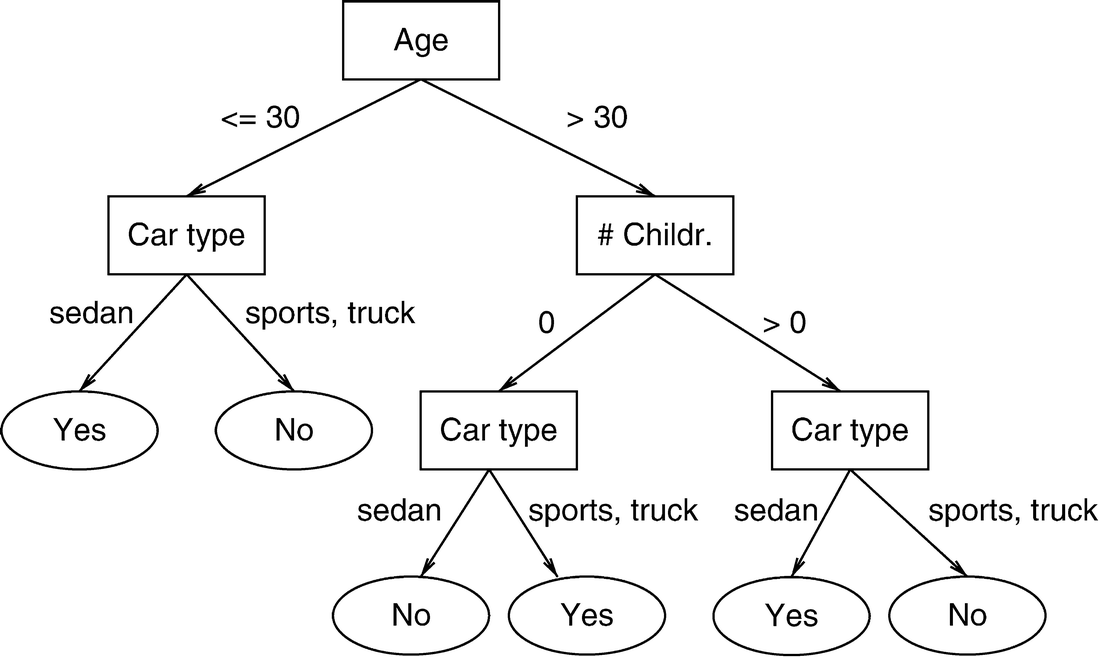Source

Hence, it is a graphical depiction of all the possible outcomes to a problem based on certain conditions or as said rules. The model is trained by creating a top-down tree and then this trained decision tree is used to test the new or the unseen data to classify these cases into a category.

It is important to note that by design the decision tree algorithm tries to build the tree where the smallest leaf nodes are homogenous in the dependent variable. Homogeneity in the target variable means that there is a record of only one type in the outcome i.e. in the leaf node, which conveys the car type is either sedan or sports truck. At times, the challenge is that the tree is restricted meaning it is forced to stop growing or the features are exhausted to use to break the branch into smaller leaf nodes, in such a scenario the objective variable is not homogenous and the outcome is still a mix of the car types.

How does a decision tree algorithm select the feature and what is the threshold or the juncture within that feature to build the tree? To answer this, we need to dig into the evergreen concept of any machine learning algorithm, yes…you guessed it right! It’s the loss function, indeed!

## Cost Function in a Decision Tree

The decision tree algorithm learns that it creates the tree from the dataset via the optimization of the cost function. In the case of classification problems, the cost or the loss function is a measure of impurity in the target column of nodes belonging to a root node.

The impurity is nothing but the surprise or the uncertainty available in the information that we had discussed above. At a given node, the impurity is a measure of a mixture of different classes or in our case a mix of different car types in the Y variable. Hence, the impurity is also referred to as heterogeneity present in the information or at every node.

The goal is to minimize this impurity as much as possible at the leaf (or the end-outcome) nodes. It means the objective function is to decrease the impurity (i.e. uncertainty or surprise) of the target column or in other words, to increase the homogeneity of the Y variable at every split of the given data.

To understand the objective function, we need to understand how the impurity or the heterogeneity of the target column is computed. There are two metrics to estimate this impurity: Entropy and Gini. In addition to this, to answer the previous question on how the decision tree chooses the attributes, there are various splitting methods including Chi-square, Gini-index, and Entropy however, the focus here is on Entropy and we will further explore how it helps to create the tree.

Now, it’s been a while since I have been talking about a lot of theory stuff. Let’s do one thing: I offer you coffee and we perform an experiment. I have a box full of an equal number of coffee pouches of two flavors: Caramel Latte and the regular, Cappuccino. You may choose either of the flavors but with eyes closed. The fun part is: in case you get the caramel latte pouch then you are free to stop reading this article 🙂 or if you get the cappuccino pouch then you would have to read the article till the end 🙂

This predicament where you would have to decide and this decision of yours that can lead to results with equal probability is nothing else but said to be the state of maximum uncertainty. In case, I had only caramel latte coffee pouches or cappuccino pouches then we know what the outcome would have been and hence the uncertainty (or surprise) will be zero.

The probability of getting each outcome of a caramel latte pouch or cappuccino pouch is:

P(Coffee pouch == Caramel Latte) = 0.50

P(Coffee pouch == Cappuccino) = 1 – 0.50 = 0.50

When we have only one result either caramel latte or cappuccino pouch, then in the absence of uncertainty, the probability of the event is:

P(Coffee pouch == Caramel Latte) = 1

P(Coffee pouch == Cappuccino) = 1 – 1 = 0

There is a relationship between heterogeneity and uncertainty; the more heterogeneous the event the more uncertainty. On the other hand, the less heterogeneous, or so to say, the more homogeneous the event, the lesser is the uncertainty. The uncertainty is expressed as Gini or Entropy.

## How does Entropy actually Work?

Claude E. Shannon had expressed this relationship between the probability and the heterogeneity or impurity in the mathematical form with the help of the following equation:

H(X) = – Σ (pi * log2 pi)The uncertainty or the impurity is represented as the log to base 2 of the probability of a category (pi). The index (i) refers to the number of possible categories. Here, i = 2 as our problem is a binary classification.

This equation is graphically depicted by a symmetric curve as shown below. On the x-axis is the probability of the event and the y-axis indicates the heterogeneity or the impurity denoted by H(X). We will explore how the curve works in detail and then shall illustrate the calculation of entropy for our coffee flavor experiment.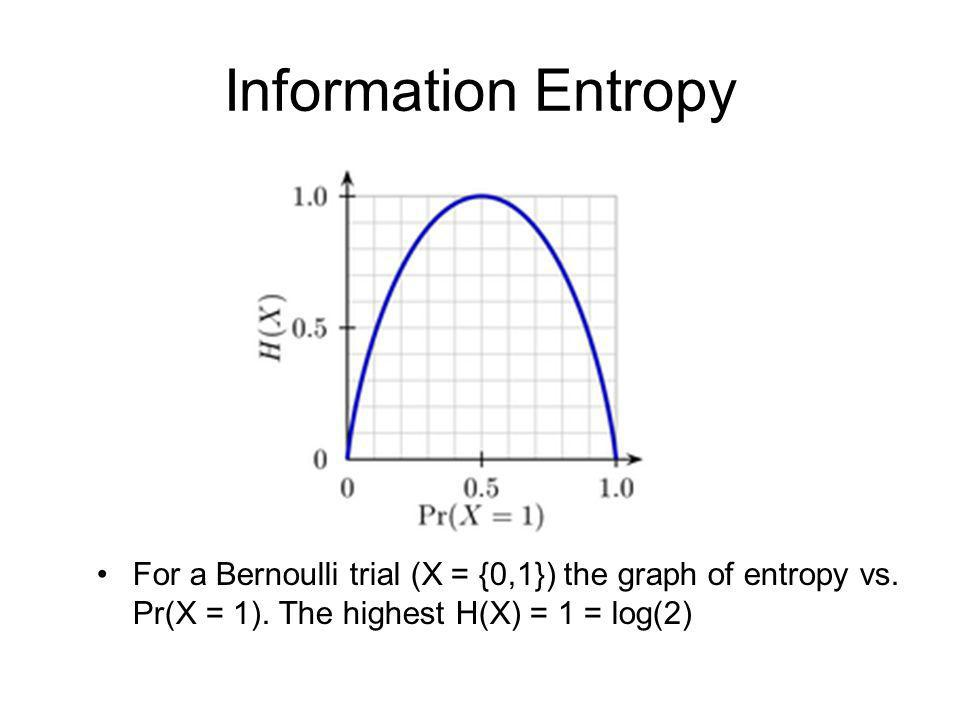The log2 pi has a very unique property that is when there are only two outcomes say probability of the event = pi is either 1 or 0.50 then in such scenario log2 pi takes the following values (ignoring the negative term):

 pi  = 1 pi = 0.50 log2 pi log2 (1) = 0 log2 (0.50) = 1

Now, the above values of the probability and log2 pi are depicted in the following manner: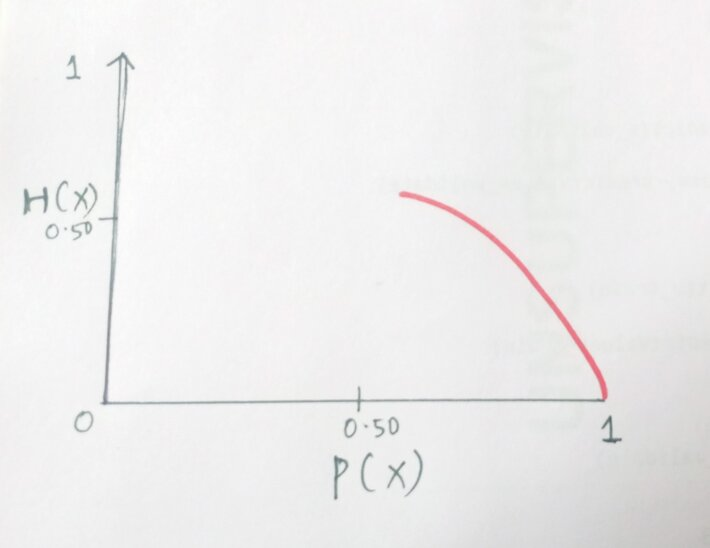The catch is when the probability, pi becomes 0, then the value of log2 p0 moves towards infinity and the curve changes its shape to: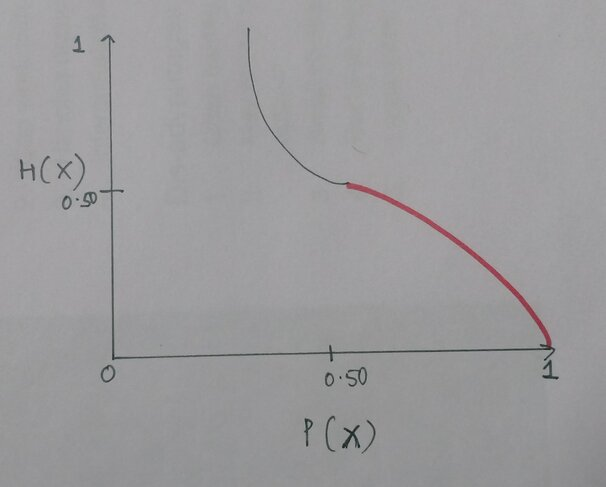The entropy or the impurity measure can only take value from 0 to 1 as the probability ranges from 0 to 1 and hence, we do not want the above situation. So, to make the curve and the value of  log2 pback to zero, we multiply log2 pwith the probability i.e. with pi itself.

Therefore, the expression becomes (pi*  log2 pi) and  log2 pi returns a negative value and to remove this negativity effect, we multiply the resultant with a negative sign and the equation finally becomes:

H(X) = – Σ (pi * log2 pi)

Now, this expression can be used to show how the uncertainty changes depending on the likelihood of an event.

The curve finally becomes and holds the following values:

This scale of entropy from 0 to 1 is for binary classification problems. For a multiple classification problem, the above relationship holds, however, the scale may change.

## Calculation of Entropy in Python

We shall estimate the entropy for three different scenarios. The event Y is getting a caramel latte coffee pouch. The heterogeneity or the impurity formula for two different classes is as follows:

H(X) = – [(pi * log2 pi) + (qi * log2 qi)]

where,

pi = Probability of Y = 1 i.e. probability of success of the event

q= Probability of Y = 0 i.e. probability of failure of the event

Case 1:

 Coffee flavor Quantity of Pouches Probability Caramel Latte 7 0.7 Cappuccino 3 0.3 Total 10 1

H(X) = – [(0.70 * log2 (0.70)) + (0.30 * log2 (0.30))] = 0.88129089

This value 0.88129089 is the measurement of uncertainty when given the box full of coffee pouches and asked to pull out one of the pouches when there are seven pouches of caramel latte flavor and three pouches of cappuccino flavor.

Case 2:

 Coffee flavor Quantity of Pouches Probability Caramel Latte 5 0.5 Cappuccino 5 0.5 Total 10 1

H(X) = – [(0.50 * log2 (0.50)) + (0.50 * log2 (0.50))] = 1

Case 3:

 Coffee flavor Quantity of Pouches Probability Caramel Latte 10 1 Cappuccino 0 0 Total 10 1

H(X) = – [(1.0 * log2 (1.0) + (0 * log2 (0)] ~= 0

In scenarios 2 and 3, can see that the entropy is 1 and 0, respectively. In scenario 3, when we have only one flavor of the coffee pouch, caramel latte, and have removed all the pouches of cappuccino flavor, then the uncertainty or the surprise is also completely removed and the aforementioned entropy is zero. We can then conclude that the information is 100% present.

Python Code:

So, in this way, we can measure the uncertainty available when choosing between any one of the coffee pouches from the box. Now, how does the decision tree algorithm use this measurement of impurity to build the tree?

## Use of Entropy in Decision Tree

As we have seen above, in decision trees the cost function is to minimize the heterogeneity in the leaf nodes. Therefore, the aim is to find out the attributes and within those attributes the threshold such that when the data is split into two, we achieve the maximum possible homogeneity or in other words, results in the maximum drop in the entropy within the two tree levels.

At the root level, the entropy of the target column is estimated via the formula proposed by Shannon for entropy. At every branch, the entropy computed for the target column is the weighted entropy. The weighted entropy means taking the weights of each attribute. The weights are the probability of each of the classes. The more the decrease in the entropy, the more is the information gained.

Information Gain is the pattern observed in the data and is the reduction in entropy. It can also be seen as the entropy of the parent node minus the entropy of the child node. It is calculated as 1 – entropy. The entropy and information gain for the above three scenarios is as follows:

 Entropy Information Gain Case 1 0.88129089 0.11870911 Case 2 1 0 Case 3 0 1

The estimation of Entropy and Information Gain at the node level:

We have the following tree with a total of four values at the root node that is split into the first level having one value in one branch (say, Branch 1) and three values in the other branch (Branch 2). The entropy at the root node is 1.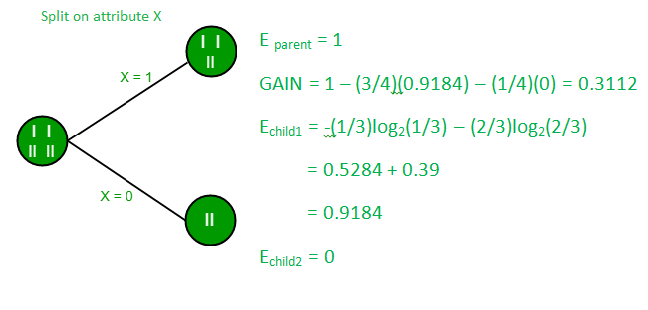Source: GeeksforGeeks

Now, to compute the entropy at the child node 1, the weights are taken as ⅓ for Branch 1 and ⅔ for Branch 2 and are calculated using Shannon’s entropy formula. As we had seen above, the entropy for child node 2 is zero because there is only one value in that child node meaning there is no uncertainty and hence, the heterogeneity is not present.

H(X) = – [(1/3 * log2 (1/3)) + (2/3 * log2 (2/3))] = 0.9184

The information gain for the above tree is the reduction in the weighted average of the entropy.

Information Gain = 1 – ( ¾ * 0.9184) – (¼ *0) = 0.3112

## Endnotes

Information Entropy or Shannon’s entropy quantifies the amount of uncertainty (or surprise) involved in the value of a random variable or the outcome of a random process. Its significance in the decision tree is that it allows us to estimate the impurity or heterogeneity of the target variable.

Subsequently, to achieve the maximum level of homogeneity in the response variable, the child nodes are created in such a way that the total entropy of these child nodes must be less than the entropy of the parent node.

### References:## 5 thoughts on "Entropy – A Key Concept for All Data Science Beginners"###### Sandip Kumar Ramji says:November 10, 2020 at 6:16 pm